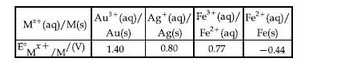# Wrong Questions in JEE Main January 2019

## 13 Questions Claimed Wrong in JEE Main 2019, Students get Bonus Marks.

The last exam of JEE Main 2019 January session was on 12th January, after that the exam conducting authority released the Question paper and Answer key. Many aspirants found some questions and answers were wrong and NTA give them an opportunity to challenge the answer key. As per NTA, If you want to challenge the answer key, you have to submit the document as a proof with a fee of rs .1000 for each question. According to our Entrance360 experts, there could be 13 Questions which were wrong, 9 Questions in Physics( That's why physics is tough), 2 Questions each in Chemistry and mathematics. In this article, we are going to discuss the correct answers solved by our experts.

Here is a list of Question, Answers that went wrong in JEE main 2019 January session exam and the solutions are provided by our experts.

Chemistry Question: 9th Jan Slot 1

0.5 moles of gas A and x moles of gas Bexert a pressure of 200 Pa in a container of volume 10m3at 1000 K.Given R is the gas constant in JK-1,x is:Physics Questions: 9th Jan Slot 2

A parallel plate capacitor with square plates is filled with four dielectrics constant K1 , K2, K3 , K4 arranged as shown in the figure. The effective dielectric constant K will be:

Correct Answer: Is not given in any of the options.SolutionMaths Question: 10th Jan Slot 1

Let  be any two non-zero complex numbers such that. If  then :

Correct Answer: Is not given in any of the options

Solution:Physics Question: 9th Jan Slot 1

A conducting circular loop made of a thin wire has area   and resistance. It is placed perpendicular to a time-dependent magnetic field  The field is uniform in space. Then the net charge flowing through the loop during  is close to :

Correct Answer: Is not given in any of the options

Solution:Physics Question: 10th Jan Slot 2

A cylindrical plastic bottle of negligible mass is filled with 310 ml of water and left floating in a pond with still water. If pressed downward slightly and released, it starts performing simple harmonic motion at angular frequency . If the radius of the bottle is 2.5 cm then   is close to : (density of water = 103 Kg/m3)

Correct Answer: Is not given in any of the options

Solution:Physics Question: 11th Jan Slot 1

A particle undergoing simple harmonic motion has a dependent displacement given by x(t) = . The ratio of kinetic to the potential energy of this particle at t = 210s will be:

Correct Answer: Is not given in any of the options

Solution:Physics Question: 11th Jan Slot 1

In the circuit shown,the switch S1 is closed at time t = 0 and the switch S2, is kept open. At some later time (t0), the switch S1 is opened and S2 is closed. The behavior of the current I as a function of time 't' is given by

Correct Answer: Is not given in any of the options
SolutionPhysics Question: 11th Jan Slot 2

The region between y = 0 and y = d contains a magnetic field . A particle of mass m and charge q enters the region with a velocity . If , the acceleration of the charged particle at the point of its emergence at the other side is:

Correct Answer: Is not given in any of the options

Solution:Chemistry Question: 11th Jan Slot 1

For the cell Zn (s), different half cells and their standerd electrode potential are given below :If which cathode will give a maximun value of   per electron transfered?

Solution:Physics question: 12th Jan Slot 2

A soap bubble, blown by a mechanical pump at the mouth of a tube, increases in volume, with time, at a constant rate. The graph that correctly depicts the time dependence of pressure inside the bubble is given by :

Correct Answer: Is not given in any of the options

SolutionPhysics Question: 11th Jan Slot 1

A body of mass 1kg falls freely from a height of 100m, on a platform of mass 3kg which is mounted on a spring having spring constant k = 1.25 X 106N/m. The body sticks to the platform and the spring's maximum compression is found to be x. Given that g = 10ms-2, the value of x will be close to:

Correct Answer: Is not given in any of the options

SolutionPhysics Question: 12th Jan Slot 2

In the above circuit, C ,   ,  and  . Current in L-R1 path is I1 and in C-R2 path it is I2 . The voltage of A.C source is given by,  volts . The phase difference between  I1 and I2 is:

Correct Answer: Is not given in any of the options

SolutionMaths Question: 11th Jan Slot 1

The direction ratios of normal to the plane through the points (0,-1,0) and (0,0,1) and making an angle   with the plane  are :

Correct Answer: Is not given in any of the options

SolutionNTA has released the final answer key on 23 Jan 2019 and makes it clear that the total of 22 questions have been dropped and full marks have been awarded to the aspirants. The authorities have also marked two or all four options as correct for some questions in the final answer key.  the NTA’s decision on the challenges shall be final and no further communication will be entertained and the result will be compiled on the basis of final answer keys. Evaluation of multiple choice questions of Paper-1 will be carried out using final answer keys and the raw (actual) marks obtained by a candidate will be considered further for computation of the result of JEE (Main)-2019.

Stay tuned with us at Entrance360 for more updates...